# Exploratory Data Analysis¶

This is complimentory tutorial for datascienceguide.github.io outlining the basics of exploratory data analysis

In this tutorial, we will learn to open a comma seperated value (CSV) data file and make find summary statistics and basic visualizations on the variables in the Ansombe dataset (to see the importance of visualization). Next we will investigate Fisher's Iris data set using more powerful visualizations.

These tutorials assumes a basic understanding of python so for those new to python, understanding basic syntax will be very helpful. I recommend writing python code in Jupyter notebook as it allows you to rapidly prototype and annotate your code.

Python is a very easy language to get started with and there are many guides: Full list: http://docs.python-guide.org/en/latest/intro/learning/

Once you are familiar with python, the first part of this guide is useful in learning some of the libraries we will be using: http://cs231n.github.io/python-numpy-tutorial

In addition, the following post helps teach the basics for data analysis in python:

We should store this in a known location on our local computer or server. The simplist way is to download and save it in the same folder you launch Jupyter notebook from, but I prefer to save my datasets in a datasets folder 1 directory up from my tutorial code (../datasets/).

You should dowload the following CSVs:

http://datascienceguide.github.io/datasets/anscombe_i.csv

http://datascienceguide.github.io/datasets/anscombe_ii.csv

http://datascienceguide.github.io/datasets/anscombe_iii.csv

http://datascienceguide.github.io/datasets/anscombe_iv.csv

http://datascienceguide.github.io/datasets/iris.csv

If using a server, you can download the file by using the following command:

wget http://datascienceguide.github.io/datasets/iris.csv


Now we can run the following code to open the csv.

In :
import matplotlib.pyplot as plt
import numpy as np
import pandas as pd
%matplotlib inline



The first three lines of code import libraries we are using and renames to shorter names.

Matplotlib is a python 2D plotting library which produces publication quality figures in a variety of hardcopy formats and interactive environments across platforms. We will use it for basic graphics

Numpy is the fundamental package for scientific computing with Python. It contains among other things:

• a powerful N-dimensional array object
• tools for integrating C/C++ and Fortran code
• useful linear algebra, Fourier transform, and random number capabilities

Pandas is open source, BSD-licensed library providing high-performance, easy-to-use data structures and data analysis tools for the Python programming language.

It extends the numpy array to allow for columns of different variable types.

Since we are using Jupyter notebook we use the line %matplotlib inline to tell python to put the figures inline with the notebook (instead of a popup)

pd.read_csv opens a .csv file and stores it into a dataframe object which we call anscombe_i, anscombe_ii, etc.

Next, let us see the structure of the data by printing the first 5 rows (using [:5]) data set:

In :
print anscombe_i[0:5]

    x     y
0  10  8.04
1   8  6.95
2  13  7.58
3   9  8.81
4  11  8.33


Now let us use the describe function to see the 3 most basic summary statistics

In :
print "Data Set I"
print anscombe_i.describe()[:3]
print "Data Set II"
print anscombe_ii.describe()[:3]
print "Data Set III"
print anscombe_iii.describe()[:3]
print "Data Set IV"
print anscombe_iv.describe()[:3]

Data Set I
x          y
count  11.000000  11.000000
mean    9.000000   7.500909
std     3.316625   2.031568
Data Set II
x          y
count  11.000000  11.000000
mean    9.000000   7.500909
std     3.316625   2.031657
Data Set III
x          y
count  11.000000  11.000000
mean    9.000000   7.500000
std     3.316625   2.030424
Data Set IV
x          y
count  11.000000  11.000000
mean    9.000000   7.500909
std     3.316625   2.030579


It appears that the datasets are almost identical by looking only at the mean and the standard deviation. Instead, let us make a scatter plot for each of the data sets.

Since the data is stored in a data frame (similar to an excel sheet), we can see the column names on top and we can access the columns using the following syntax

anscombe_i.x

anscombe_i.y



or

anscombe_i['x']

anscombe_i['y']
In :
plt.figure(1)
plt.scatter(anscombe_i.x, anscombe_i.y,  color='black')
plt.title("anscombe_i")
plt.xlabel("x")
plt.ylabel("y")
plt.figure(2)

plt.scatter(anscombe_ii.x, anscombe_ii.y,  color='black')
plt.title("anscombe_ii")
plt.xlabel("x")
plt.ylabel("y")

plt.figure(3)
plt.scatter(anscombe_iii.x, anscombe_iii.y,  color='black')
plt.title("anscombe_iii")
plt.xlabel("x")
plt.ylabel("y")

plt.figure(4)
plt.scatter(anscombe_iv.x, anscombe_iv.y,  color='black')
plt.title("anscombe_iv")
plt.xlabel("x")
plt.ylabel("y")

Out:
<matplotlib.text.Text at 0x7f9a23c05350>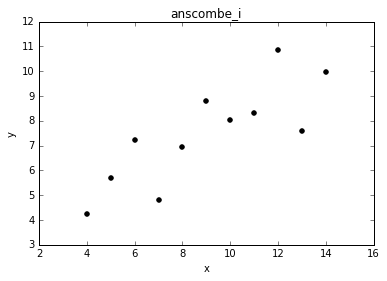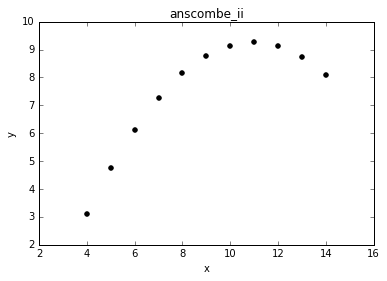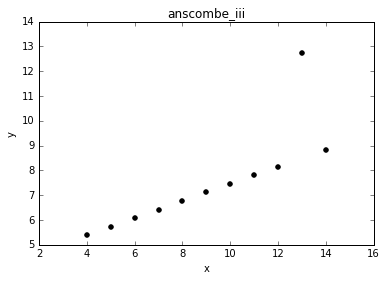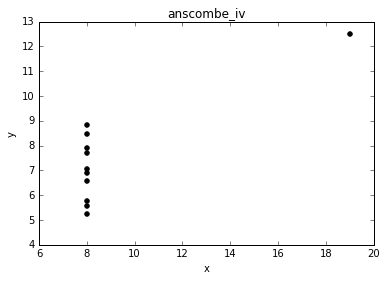Shockily we can clearly see that the datasets are quite different! The first data set has pure irreducable error, the second data set is not linear, the third dataset has an outlier, and the fourth dataset all of x values are the same except for an outlier. If you do not believe me, I uploaded an excel worksheet with the full datasets and summary statistics here

Now let us learn how to make a box plot. Before writing this tutorial I didn't know how to make a box plot in matplotlib (I usually use seaborn which we will learn soon). I did a quick google search for "box plot matplotlib) and found an example here which outlines a couple of styling options.

In :
# basic box plot
plt.figure(1)
plt.boxplot(anscombe_i.y)
plt.title("anscombe_i y box plot")

Out:
<matplotlib.text.Text at 0x7f9a23a74e50>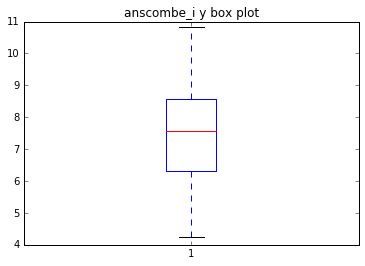Trying reading the documentation for the box plot above and make your own visuaizations.

Next we are going to learn how to use Seaborn which is a very powerful visualization library. Matplotlib is a great library and has many examples of different plots, but seaborn is built on top of matplot lib and offers better plots for statistical analysis. If you do not have seaborn installed, you can follow the instructions here: http://stanford.edu/~mwaskom/software/seaborn/installing.html#installing . Seaborn also has many examples and also has a tutorial.

To show the power of the library we are going to plot the anscombe datasets in 1 plot following this example: http://stanford.edu/~mwaskom/software/seaborn/examples/anscombes_quartet.html . Do not worry to much about what the code does (it loads the same dataset and changes setting to make the visualization clearer), we will get more experince with seaborn soon.

In :
import seaborn as sns
sns.set(style="ticks")

# Load the example dataset for Anscombe's quartet

# Show the results of a linear regression within each dataset
sns.lmplot(x="x", y="y", col="dataset", hue="dataset", data=df,
col_wrap=2, ci=None, palette="muted", size=4,
scatter_kws={"s": 50, "alpha": 1})

/usr/local/lib/python2.7/dist-packages/matplotlib/__init__.py:872: UserWarning: axes.color_cycle is deprecated and replaced with axes.prop_cycle; please use the latter.
warnings.warn(self.msg_depr % (key, alt_key))

Out:
<seaborn.axisgrid.FacetGrid at 0x7f9a23a8f910>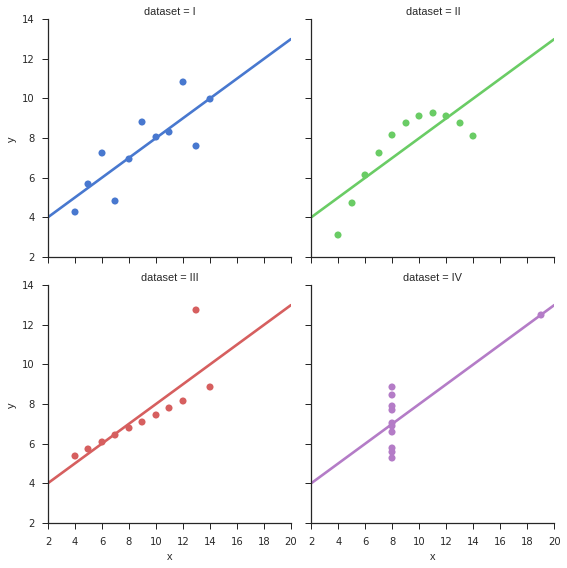Seaborn does linear regression automatically (which we will learn soon). We can also see that the linear regression is the same for each dataset even though they are quite different.

The big takeway here is that summary statistics can be deceptive! Always make visualizations of your data before making any models.

# Irist Dataset¶

Next we are going to visualize the Iris dataset. Let us first read the .csv and print the first elements of the dataframe. We also get the basic summary statistics.

In :
iris = pd.read_csv('../datasets/iris.csv')
print iris[0:5]

print iris.describe()

   sepal length  sepal width  petal length  petal width         iris
0           5.1          3.5           1.4          0.2  Iris-setosa
1           4.9          3.0           1.4          0.2  Iris-setosa
2           4.7          3.2           1.3          0.2  Iris-setosa
3           4.6          3.1           1.5          0.2  Iris-setosa
4           5.0          3.6           1.4          0.2  Iris-setosa
sepal length  sepal width  petal length  petal width
count    150.000000   150.000000    150.000000   150.000000
mean       5.843333     3.054000      3.758667     1.198667
std        0.828066     0.433594      1.764420     0.763161
min        4.300000     2.000000      1.000000     0.100000
25%        5.100000     2.800000      1.600000     0.300000
50%        5.800000     3.000000      4.350000     1.300000
75%        6.400000     3.300000      5.100000     1.800000
max        7.900000     4.400000      6.900000     2.500000


As we can see, it is difficult to interpret the results. We can see that sepal length, sepal width, petal length and petal width are all numeric features, and the iris variable is the specific type of iris (or categorical variable). To better understand the data, we can split the data based on each type of iris, make a histogram for each numeric feature, scatter plot between features and make many visualizations. I will demonstrate the process for generating a histogram for sepal length of Iris-setosa and a scatter plot for sepal length vs width for Iris-setosa

In :
#select all Iris-setosa
iris_setosa = iris[iris.iris == "Iris-setosa"]
plt.figure(1)
#make histogram of sepal lenth
plt.hist(iris_setosa["sepal length"])
plt.xlabel("sepal length")

plt.figure(2)
plt.scatter(iris_setosa["sepal width"], iris_setosa["sepal length"] )
plt.xlabel("sepal width")
plt.ylabel("sepal lenth")

Out:
<matplotlib.text.Text at 0x7f9a1a4d73d0>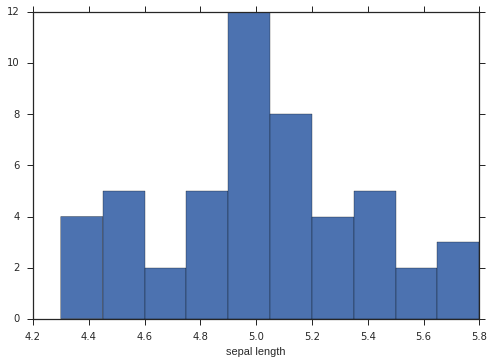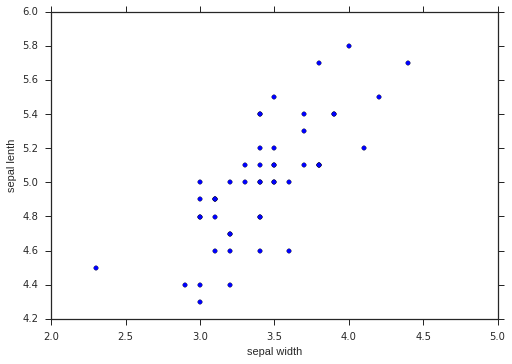This would help us to better undestand the data and is necessary for good analysis, but to do this for all the features and iris types (classes) would take a significant amount of time. Seaborn has a function called the pairplot which will do all of that for us!

In :
sns.pairplot(iris, hue="iris")

/usr/local/lib/python2.7/dist-packages/matplotlib/__init__.py:892: UserWarning: axes.color_cycle is deprecated and replaced with axes.prop_cycle; please use the latter.
warnings.warn(self.msg_depr % (key, alt_key))

Out:
<seaborn.axisgrid.PairGrid at 0x7f9a1a36f150>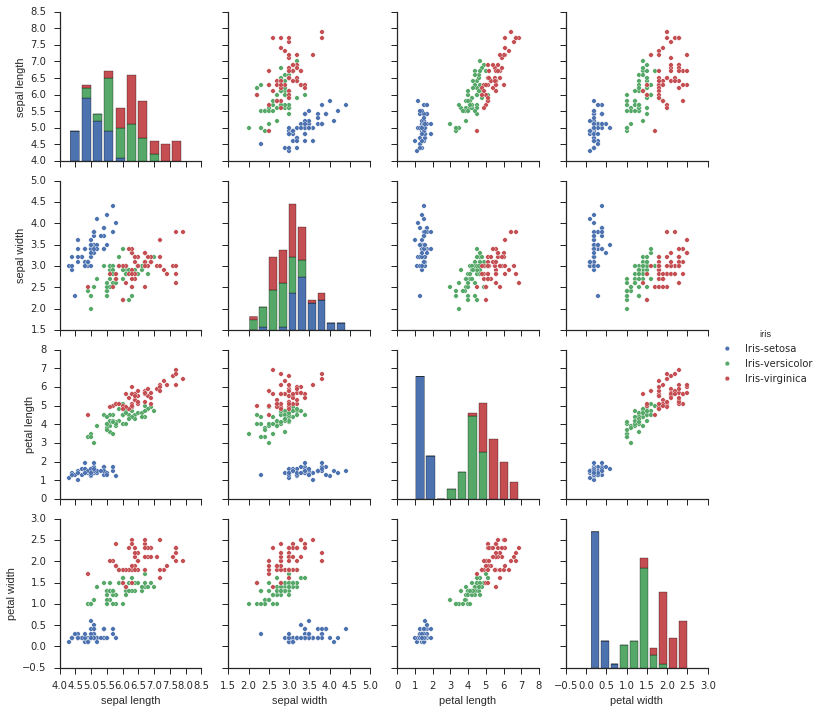We have a much better understanding of the data. For example we can see linear correlations between some of the numeric features. We can also see which numeric features seperate seperate the types of iris well and which would not.

Exploratory data analysis is not done! We could spend a whole course on doing exploratory data analysis (I took one when I was on exchange at the National Univesity of Singapore). For this reason, EDA will be a re-occuring theme in these tutorials and we will continue to always visualizate data. Data will come in different forms, it is our role as data scientists to quickly and effectively understand data.

In the next tutorial we will be using the ansombe dataset for regression, and in future tutorials we will re-visting the iris dataset to do classification.

# Next Actions:¶

Exploratory data analysis is always an ongoing process, and we we have learnt in this tutorial, it is a necessary step before we start modeling. The way to get better at plotting data is to get started plotting! Pick an interesting dataset you can find and start exploring!

Here a some datasets to get you started:

You can also look for examples and sample code online for others using matplotlib, seaborn and ggplot2 (for those using R) for inspiration.

Have fun!